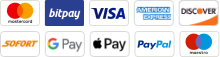# An Insightful Comparison of Mathematicians and Statisticians

In the huge realm of numbers, patterns, and data, two prominent figures emerge: mathematicians and statisticians. Their approaches, applications, and ultimate goals can be distinctively different. Due to this, it is crucial to understand what both kinds of individuals do if you are considering a master of statistics online. Today, we shall look at each in proper detail.

## Mathematicians Vs. Statisticians

### Focus

Mathematician: Often concentrates on developing abstract theories and solving theoretical problems using mathematical methods.

Statistician: Focuses on making sense of data. They use mathematical techniques to analyze and interpret data and draw conclusions.

### Applications

Mathematician: Their work can be applied in physics, engineering, economics, and even in abstract mathematical theories.

Statistician: Commonly work in fields like economics, biology, health sciences, politics, and any other field where data interpretation is crucial.

### Tools

Mathematician: Uses mathematical theories, theorems, and formulas.

Statistician: Uses tools like standard deviation, mean, median, probability theories, and software specific for data analysis like R or SPSS.

### Objective

Mathematician: To solve problems and establish truths using logical reasoning and mathematical proof.

Statistician: To derive meaningful insights from data and predict future trends or outcomes.

### Work Environment

Mathematician: Often found in academia, research institutions, or specialized industries requiring deep mathematical knowledge.

Statistician: Work in various industries including government, healthcare, sports, marketing, and any field requiring data analysis.

## Which One to Opt for As a Career Path

### Nature of Work

Mathematician: Focuses on theoretical or applied mathematics, solving problems, and developing theories using mathematical methods. They often work in academia, research, or specialized industries like cryptography or theoretical physics.

Statistician: Uses statistical methods to collect, analyze, interpret, and present data. They often work in various sectors like healthcare, economics, biology, and public policy, helping make informed decisions based on data.

### Skills Required

Mathematician: Strong analytical thinking, abstract reasoning, and a deep understanding of mathematical theories and principles.

Statistician: Analytical skills combined with the ability to interpret and visualize data, proficiency in statistical software, and often domain-specific knowledge.

### Job Market And Opportunities

Mathematician: Generally, fewer positions, especially in pure mathematics, but those with applied mathematics skills can find opportunities in engineering, computer science, and data science fields.

Statistician: As data becomes increasingly crucial, the demand for statisticians is rising, especially in sectors like biostatistics, finance, and government.

### Interests

Mathematician: If you enjoy abstract thinking, proving theorems, or solving non-standard problems using mathematics, this might be for you.

Statistician: If you like working with real-world data, interpreting results, and helping make decisions based on data, consider this path.

### Educational Path

Statistician: Many positions are available with a master’s degree, though a Ph.D. might be required for research or higher-level roles.

### Collaboration

Mathematician: Might work independently, especially in theoretical fields.

Statistician: Often collaborates with other professionals, interpreting data for practical applications.

## Endnote

Both mathematicians and statisticians are essential in deepening our grasp of the world using numerical insights and patterns. Whether they operate independently or collaboratively, their contributions are crucial to the continuous unfolding of understanding and exploration.

September 22, 2023## Payment methods possible